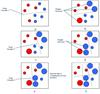adaboost 订阅
Adaboost是一种迭代算法，其核心思想是针对同一个训练集训练不同的分类器(弱分类器)，然后把这些弱分类器集合起来，构成一个更强的最终分类器（强分类器）。 展开全文Adaboost是一种迭代算法，其核心思想是针对同一个训练集训练不同的分类器(弱分类器)，然后把这些弱分类器集合起来，构成一个更强的最终分类器（强分类器）。

Adaboost

Adaboost

adaboost简介
Boosting，也称为增强学习或提升法，是一种重要的集成学习技术，能够将预测精度仅比随机猜度略高的弱学习器增强为预测精度高的强学习器，这在直接构造强学习器非常困难的情况下，为学习算法的设计提供了一种有效的新思路和新方法。作为一种元算法框架，Boosting几乎可以应用于所有目前流行的机器学习算法以进一步加强原算法的预测精度，应用十分广泛，产生了极大的影响。而AdaBoost正是其中最成功的代表，被评为数据挖掘十大算法之一。在AdaBoost提出至今的十几年间，机器学习领域的诸多知名学者不断投入到算法相关理论的研究中去，扎实的理论为AdaBoost算法的成功应用打下了坚实的基础。AdaBoost的成功不仅仅在于它是一种有效的学习算法，还在于1)它让Boosting从最初的猜想变成一种真正具有实用价值的算法；2)算法采用的一些技巧，如:打破原有样本分布，也为其他统计学习算法的设计带来了重要的启示；3)相关理论研究成果极大地促进了集成学习的发展。 

• ## Adaboost

千次阅读 2016-04-16 21:07:14
Adaboost
Adaboost

Adaboost算法是将弱分类器线性叠加为一个强分类器，具体的算法步骤如下：
1. 读取数据集X（X1…Xn）以及其标签Y
2. 初始化样本的权值w=1/n，设定弱分类器个数T
3. for i=1…T
对每一个特征训练一个弱分类器，将误差最小的压入弱分类器中；
计算权重误差函数e=∑i=1nwiI(h(xi)≠yi)<!--//--><![CDATA[//><!--
e = \sum\limits_{i = 1}^n {w_i I(h(xi) \ne yi)}
//--><!]]>
计算分类器权重α=0.5ln(1−ee)<!--//--><![CDATA[//><!--
\alpha  = 0.5\ln \left( {\frac{{1 - e}}{e}} \right)
//--><!]]>
更新样本权值w=wsum(w)×{e−αh(xi)=yieαh(xi)≠yi<!--//--><![CDATA[//><!--
w = \frac{w}{{sum\left( w \right)}} \times \left\{ \begin{array}{l}
e^{ - \alpha } h(xi) = yi \\
e^\alpha  h(xi) \ne yi \\
\end{array} \right.
//--><!]]>
4. 预测结果：H(x)=sign(∑i=1Tαihi(x))<!--//--><![CDATA[//><!--
H\left( x \right) = sign\left( {\sum\limits_{i = 1}^T {\alpha _i h_i (x)} } \right)
//--><!]]>参照这个地方的代码写的，谢谢作者，写的很清楚c++源码

Adaboost优点：
低泛化误差，分类准确率高，不用担心过拟合。
缺点：
对离群点比较敏感。
展开全文• AdaBoost AdaBoost是典型的Boosting算法，即找到相对容易的弱学习算法，然后通过反复学习得到一系列弱分类器，组合这些弱分类器得到一个强分类器。Boosting算法要涉及到两个部分，加法模型和前向分步算法。 加法...
AdaBoost
AdaBoost是典型的Boosting算法，即找到相对容易的弱学习算法，然后通过反复学习得到一系列弱分类器，组合这些弱分类器得到一个强分类器。Boosting算法要涉及到两个部分，加法模型和前向分步算法。

加法模型就是说强分类器由一系列弱分类器线性相加而成。
前向分步就是说在训练过程中，下一轮迭代产生的分类器是在上一轮的基础上训练得来的。

由于采用的损失函数不同，Boosting算法也因此有了不同的类型，AdaBoost就是损失函数为指数损失的Boosting算法
流程
输入：训练数据集$T=\{(x_1,y_1),(x_2,y_2),(x_N,y_N)\}$，其中，$x_i∈X⊆R^n$，$y_i∈Y={-1,1}$，迭代次数M
a. 初始化训练样本的权值分布：$D_1=(w_{1,1},w_{1,2},…,w_{1,i}),w_{1,i}=\frac{1}{N},i=1,2,…,N$
b. 对于$m=1,2,…,M$

使用具有权值分布$D_m$的训练数据集进行学习，得到弱分类器$G_m(x)$
计算$G_m(x)$在训练数据集上的分类误差率$e_m=\sum_{i=1}^Nw_{m,i} I(G_m (x_i )≠y_i )$
计算$G_m(x)$在强分类器中所占权重$α_m=\frac{1}{2}log \frac{1-e_m}{e_m}$
更新训练数据集的权值分布，$z_m$是归一化因子，可将样本概率分布和为1$w_{m+1,i}=\frac{w_{m,i}}{z_m}exp⁡(-α_m y_i G_m (x_i ))，i=1,2,…,10$
$z_m=\sum_{i=1}^Nw_{m,i}exp⁡(-α_m y_i G_m (x_i ))$

c. 最终分类器
$F(x)=sign(\sum_{i=1}^Nα_m G_m (x))$
证明与推导
adaboost的损失函数为指数函数：
$L(y,f(x))=exp[-yf(x)]$
对于分类模型而言，上述损失函数，在分类正确的时候，指数部分为负数；在分类错误的时候，指数部分为正数，符合损失函数的意义。
前向分步为：$f_m(x)=f_{m-1}(x)+\alpha_mG_m(x)$
将前向分步代入损失函数，可得：
$Loss=\sum_{i=1}^Nexp⁡(-y_i f_m (x_i ))=\sum_{i=1}^Nexp⁡(-y_i (f_{m-1} (x_i )+α_m G_m (x_i )))$此时$f_{m-1}(x)$已知，即：
$Loss=\sum_{i=1}^N\widetilde{w_{m,i}} exp⁡(-y_i α_m G_m (x_i ))$$\widetilde{w_{m,i}}=exp⁡(-y_i (F_{m-1} (x)))$
于是分类器$G_m(x)$和这个分类器的权重 $\alpha_m$ 可以表示成：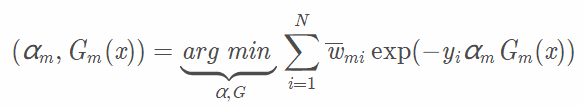先求$G_m(x)$，分类器的权重可以认为是一个确定的数，$G_m(x)$是使得分错的（带权重的）样本里损失函数最小的那个，可以写成：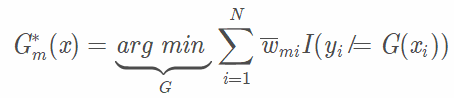得到$G^*_m(x)$以后，求$\alpha^*_m$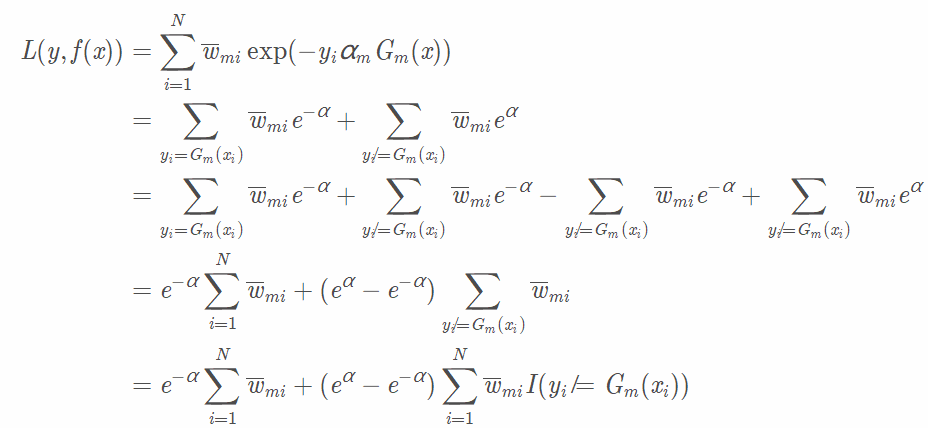把上式对  α 求导，再令导函数为 0 ，得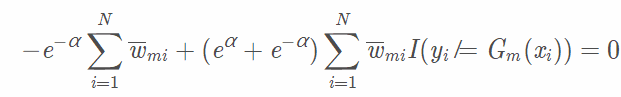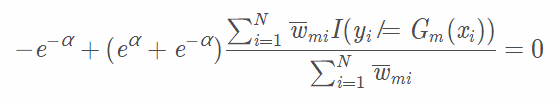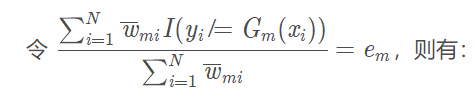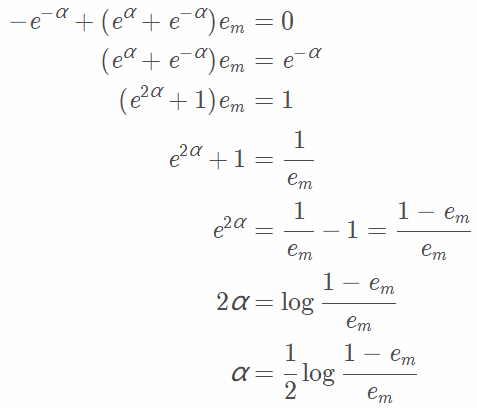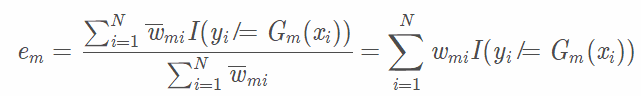更新权重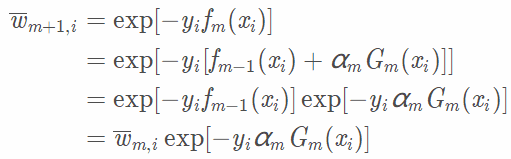相关考点

Adaboost 由于采用boosting算法，而Boosting 算法会倾向于一直分错的样本，如果样本中有离群的错误样本，boosting就会出现效果不好的情况。即Adaboost对离群值、异常值敏感。
Adaboost和GBDT的异同点
（1)   关注点：分错权值，残差：Adaboost每轮学习的一个基本学习器是通过改变样本的权值，关注上轮分类错误的样本的权值，以逐步减少在训练集上的分类误差率。而GBDT每轮学习一个基本学习器是通过改变输出值，每轮拟合的值为真实值与已有的加法模型的差值（即残差）。
（2)   异常点: adaboost存在异常点敏感的问题,  gbdt一定程度上优化了adaboost异常点敏感的问题，但是存在难以并行的缺点
（3）树: GBDT无论是进行分类还是回归问题，都用的CART回归树，adaboost对分类问题用二叉分类树，回归问题用二叉回归树。
（4）方差偏差: 两者的目标都是优化偏差bias，必然导致训练出来的数据方差var的不稳定

实战
https://blog.csdn.net/FontThrone/article/details/78834807
https://louisscorpio.github.io/2017/11/28/%E4%BB%A3%E7%A0%81%E5%AE%9E%E6%88%98%E4%B9%8BAdaBoost/


展开全文机器学习...